# Holidays

Calculate how many hours take holidays, if take 5 weeks.

Result

x =  840 h

#### Solution:

$x = 7 \cdot \ 24 \cdot \ 5 = 840 = 840 \ \text { h }$Our examples were largely sent or created by pupils and students themselves. Therefore, we would be pleased if you could send us any errors you found, spelling mistakes, or rephasing the example. Thank you!

Leave us a comment of this math problem and its solution (i.e. if it is still somewhat unclear...):Be the first to comment!## Next similar math problems:

1. A trip to the peak Dry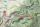Calculate what percentage is rising trip from Strečno to peak Suchý (Dry peak) longer than the descent back. Team trio of tourists (Palo, Peter and Andrew) rise took 4:21 and descent 3:08.
2. Hours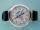How many hours is 9 days?
3. The machineThe machine works 7 hours a day and produces 1 part in 5 minutes. How many parts will it produce in 1 hour? How many parts will it produce in 1 day?
4. Rolls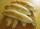Michail likes rolls, which cost 12 cents. Previously purchased 6 rolls. How many cents paid Michail for rolls?
5. Parking field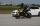There were nine cars in the parking field. The motorcycles were three times less. How many motorcycles are here and how many wheels are in the parking field?
6. Fishing boat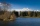The fishing boat caught 14 fish in one day. How many fish will catch 4 fishing boats in 8 days?
7. Multiples of int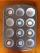How many multiples of 3 is between the numbers to 20? (zero not count)
8. Book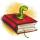Louise read from their favorite book for three days 18 pages. Every day read the same number of pages. How many pages she read every day?
9. Car parking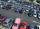The car park has 80 cars when 53 cars leaves. How many car are there?
10. Pitcher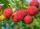Matthew picked up half-pitcher of raspberry in 45 minutes. Find how long three children filled the pitcher if each of them working at the same pace as Matthew.
11. Fridays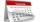Friday 13th is in 4 days. What day is today and what day it is?
12. Number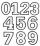Which number is 17 times larger than the number 6?
13. MultiplesFind all multiples of 10 that are larger than 136 and smaller than 214.
14. Unknown number 2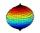I think the number. When he reduces it four times, I'll get 11. What number am I thinking?
15. Two numbersFind the four times smaller number for numbers 12 and 36 and then add them.
16. Zucchini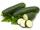One zucchini costs 5 CZK. How much would cost four?
17. Unknown number 22I am a two-digit number. My tens digits is 4. My ones digit is 3 more than my tens. Who am I?